# Training

## Training

Before an ANN can be used for any practical purpose an ANN must be trained. The training is a process during which the weights are adjusted to reach some desired goal.

 Tip Even though it is possible to adjust the weights of an ANN, it is not something typical or recommended. Instead the weights must be adjusted automatically to solve the problem at hand. For instance, humans do not enter inside another human brain to train it. Humans learns by receiving training during which little by little, they are capable of performing specific activities or they are able to solve some kind of problems.

## Training Set

Generally speaking, ANNs learning is performed using a data set. The training set is used on a new ANN (as its name indicates) for training. When humans take a class to learn a given skill, the professor organize the learning process in lessons. In the same way, the training of an ANN is organized in cases called training cases.

 Tip The training set must include as many training cases (class lessons) as possible. The set of cases must represent fully the problem at hand. It is not useful to repeat the same case several times. Instead, each case must be different.

## Training Set Components

The training set has two parts: the input and the target. The training set input contains the set of inputs that must be applied to the network. The training set target includes the set of desires values at the output of the ANN when each of the inputs specified in the training set input is applied.

 Tip The training set is usually stored in two files. Both files have the same number of rows because each row is a training case with its input and its target. The number of columns in the training set input must be equal to the number of inputs in the ANN. In the same way, the number of columns in the training set target must be equal to the number of outputs in the ANN. The format in the file is typically a comma separated file with extension *.csv. These files can be produced and read by standard software like Microsoft Excel, Matlab and Neural Lab. The figure shown below illustrates the structure of both the training set input and the training set target.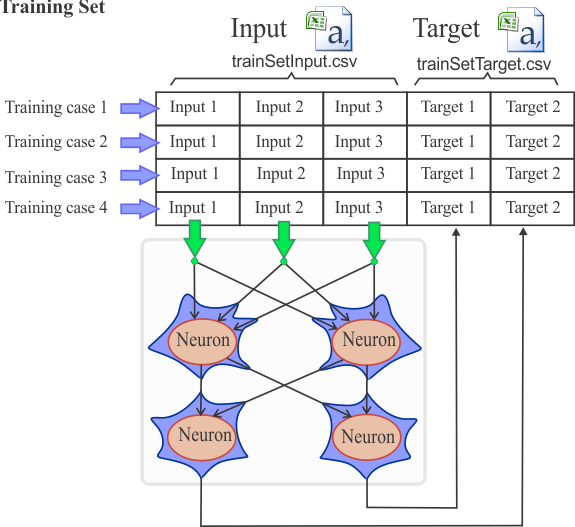Problem 1 Use Neural Lab to build the training set input for the ANN shown below. Assume that the ANN will be used to perform the logic operation AND with the ideal logic levels of 0 for false and 1 for true.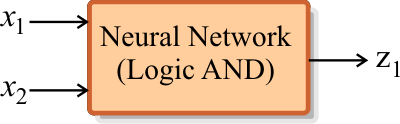Solution 1 The ANN has two inputs, x1 and x2. Because there are two input and two logic values, there are four different training cases.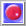Neural Lab open Neural Lab and create a new project called BuildTrainSet (use the Main file only option). The code begins by creating a matrix with four rows and two columns.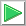Run press the button to execute the code. You will be asked to save the file, choose an appropriate folder and file name. If you do not have any errors, the variable trainSetIdeaInput will be displayed in the variable list and also on the file list. Click on the variable to see the training set input.

 BuildTrainSet\Main.lab Matrix trainSetIdeInput; trainSetIdeInput.Create(4, 2); //_______________________ row 0 (training case 0) trainSetIdeInput = 0; trainSetIdeInput = 0; //_______________________ row 1 (training case 1) trainSetIdeInput = 0; trainSetIdeInput = 1; //_______________________ row 2 (training case 2) trainSetIdeInput = 1; trainSetIdeInput = 0; //_______________________ row 3 (training case 3) trainSetIdeInput = 1; trainSetIdeInput = 1; // trainSetIdeInput.Save();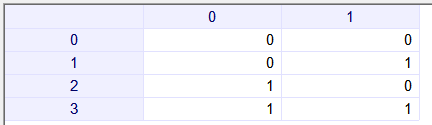Problem 2 From a practical point of view, the logic value of false can be any value between 0 and 0.2 while the value of true can be any value between 0.8 and 1.0. Build the suitable training set input that can be used in real life applications.

 Solution 2 As before, there are four different ideal training cases. However, we will represent the logic value of false for any number between 0 and 0.2. Similarly, the logic value of true will be represented by any number between 0.8 and 1.0. If we wish to include 16 times each of the four ideal training cases, the training set should include 64 training cases or rows.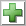Add File add a new file called BuildInputTS. The code begins by creating a matrix with 64 rows and two columns with random values from 0.0 to 2.0. The ideal values are produced using the floor function that approximates to 0 any value from 0.0 to 1.0, and to 1 any value from 1.0 to 2.0. Next, the code creates some noise. The training set input is produced by combining the ideal values with the noise.Run click the button to execute the code. You will be asked to save the file, choose an appropriate folder and file name. If you do not have any errors, the variable trainSetInput will be displayed in the variable list and also on the file list. Click on the variables to analyze your results.

 BuildTrainSet\BuildInputTS.lab Matrix noise; noise.CreateRandom(64, 2, 0, 2); // Random values in [0 2) // Matrix idealInput= floor(noise); // Round any value in [0 1) to 0 and any value in [1 2) to 1 // noise.CreateRandom(64, 2, 0, 1); //Random values in [0 1) // Matrix trainSetInput= 0.8*idealInput + 0.2*noise; trainSetInput.Save();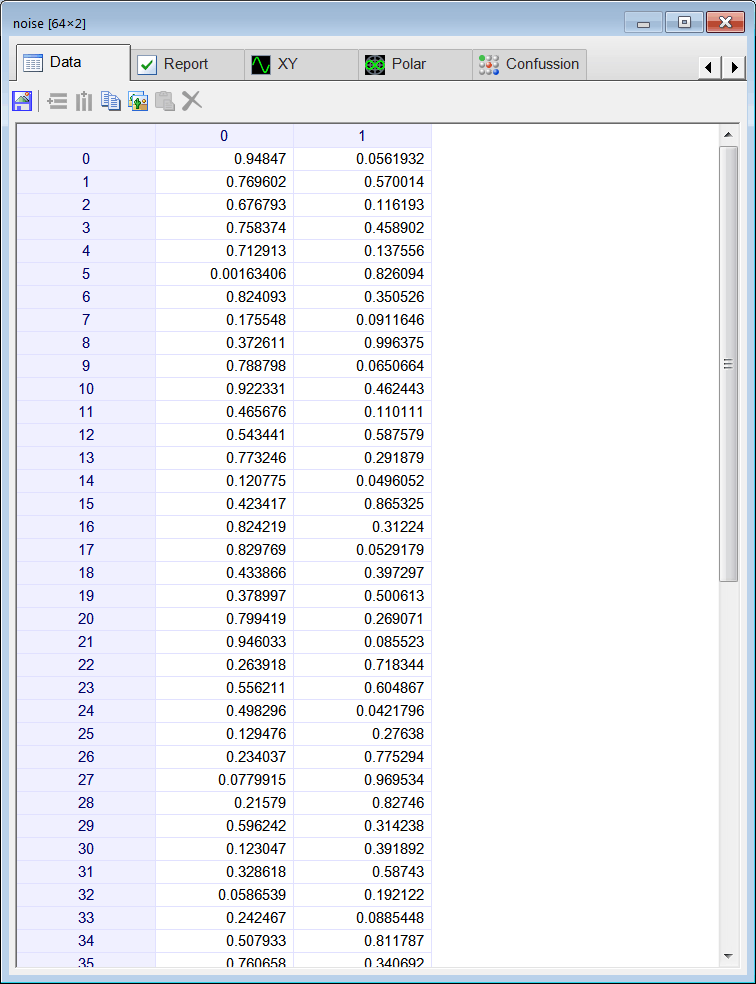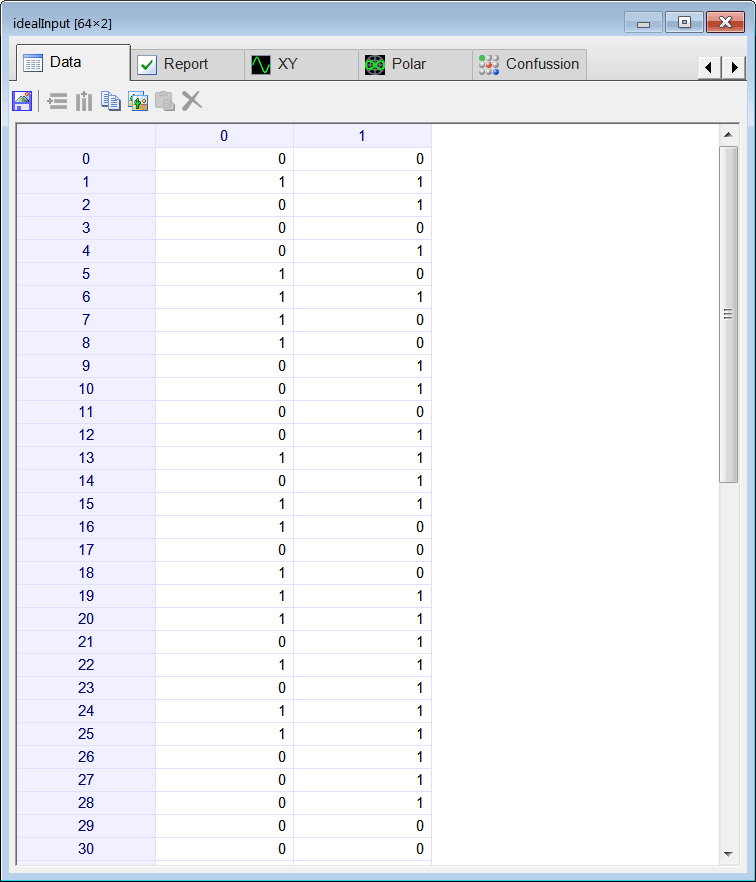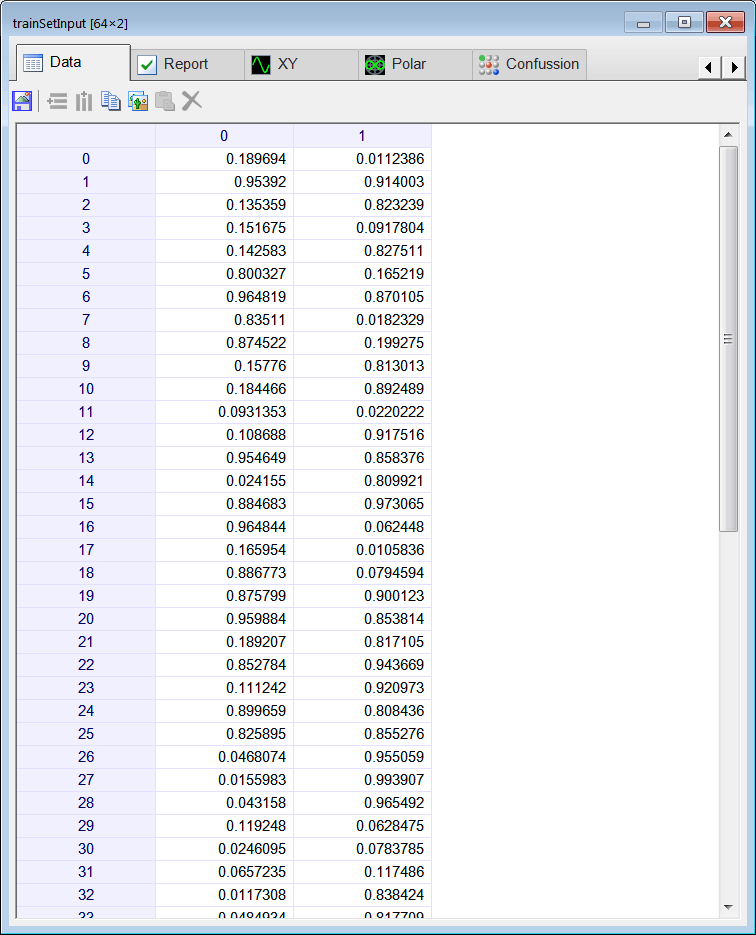Tip When combining ideal data with noise, it is very important to remember that both of them have the same range (minimum value and maximum value).

 Problem 3 A student wrote some code to build a training set input for the logic operation AND. The student insists that he can use the same code without any changes to build the training set input for the logic operations OR and XOR. Indicate whether the student is right or not.

 Problem 4 An ANN has three inputs and two outputs. A student has decided to include only one hidden layer and five neurons in this layer. If each weight needs 8 bytes for storage, compute the total number of bytes required to build the network.

 Problem 5 A researcher is using an ANN to distinguish between the letters A and B. After the preprocessing each letter can be represented by 8 numeric values. If one of the output neurons is specialized to identify the letter A, and the other output neuron is specialized to identify the letter B, how many weights should the network include? Suppose that the researcher is using 6 neurons in the first hidden layer, and 4 in the second hidden layer.

 Tip Most problems can be solved using only one hidden layer. The number of neurons in the hidden layer is a function of the number of training cases. From a practical point of view, the number of training cases must be:If the network has no hidden layers, then #traincases must be bigger than (2.2)(#outuput weights), If the network has one hidden layer, then #traincases must be bigger than (2.2)(#hidden weights). If the network has two hidden layers, then #traincases must be bigger than (2.2)(#hidden weights).

 Problem 6 A student has designed an ANN for learning of the sine and cosine functions. The ANN has one input, the angle x in radians, and two outputs: z1 = sin(x) and z2 = cos(x). The professor suggested using one hidden layer with 14 neurons in this layer. Compute the minimum number of training cases for this ANN.

 Problem 7 An experiment was designed to train an ANN with 10 inputs and 5 outputs. If the training set has 1024 training cases, compute the maximum number of neurons in the hidden layer. Suppose that the network has only one hidden layer.

 Problem 8 Repeat the last problem using Neural Lab.

 Solution 8Neural Lab open Neural Lab, create a New Project called NumTrainCases, and then write the code show below.Run click the button to execute the code. You will be asked to save the file, choose an appropriate folder and file name. If you do not have any errors, the variable numNeurons will be displayed in the variable list.

 NumTrainCases\Main.lab int numCases = 0; int numNeurons = 0; LayerNet net; while (numCases < 1024) {      numNeurons = numNeurons+1;      net.Create(10, numNeurons, 0, 5);      numCases = net.GetMinNoTrCs();      } numNeurons = numNeurons - 1;

 Problem 9 Discuss the code of the last problem.

## Training methods

There are several training methods. The most popular are:
• The method of simulated annealing
• The method of genetic algorithms
• The method of conjugate gradient
• The method of variable metric
• The method of Levenberg Marquardt
• Use regression (use the inverse of the activation function to create a set of linear equations)

 Problem 10 Search the Internet about the method of conjugate gradient. Write a one page report about it.

 Problem 11 Search the Internet about the method of Levenberg Marquardt. Write a one page report about it.